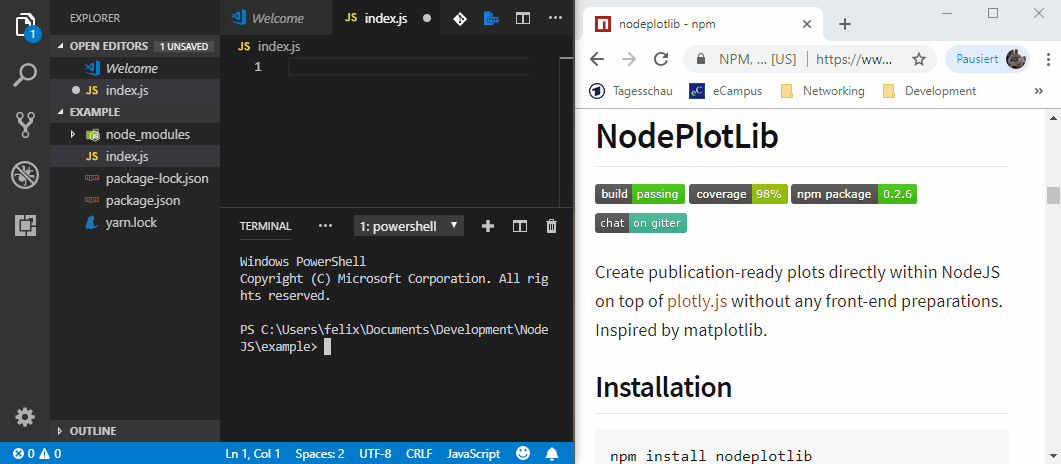# NodeJS frontend-less plotting lib using plotly.jsLibrary to create top-notch plots directly within NodeJS on top of plotly.js without any front-end preparations. Inspired by matplotlib

## NodePlotLib

Library to create top-notch plots directly within NodeJS on top of plotly.js without any front-end preparations. Inspired by matplotlib.### Installation

``````npm install nodeplotlib
# or

### Usage

#### Overview

Use with TypeScript/JavaScript:

``````import { plot, Plot } from 'nodeplotlib';
const data: Plot[] = [{x: [1, 3, 4, 5], y: [3, 12, 1, 4], type: 'line'}];
plot(data);``````

If ES5 use `require()` instead of `import`. Here is a short animation about howto and the results.

#### Detailed usage

Since Python provides with matplotlib a library for spawning plot windows, NodeJS isn't by default. But there are awesome plotting libraries for usage in front-end. So this lib targets people like scientists who easily want to create beautiful plots in a time-saving way.

The library provides a simple interface with (for now) just three functions. A `plot`, `stack` and a `clear` function. The `plot()` functions spawns a plot to the browser, if a plotdata is given as an argument. Otherwise it plots all the `stack()`ed plotdata. The arguments are of type `Plot`, which is a partial of plotly's `PlotData` type. With the `clear()` function the stack container can be cleared.

With the stack function the user is able to print multiple charts on one page.

``````import { plot, stack, clear, Plot } from 'nodeplotlib';

const data: Plot[] = [{
x: [ 1, 3, 4, 6, 7],
y: [ 2, 4, 6, 8, 9],
type: 'scatter'
}];

stack(data);
stack(data);
stack(data);
plot();``````

The plot function plots all stacked plots and the plot given by parameter (if there is one). Afterwards the temporary container gets cleared and you can call `stack()` and `plot()` again without any predefined plots.

The functions are of the form:

``````import { plot, stack, clear, Plot, Layout } from 'nodeplotlib';

plot(data?: Plot[], layout?: Layout): void;
stack(data: Plot[], layout?: Layout): void;
clear(): void;``````

### Quick start

In this section there are some examples to getting started. See the full plotly cheatsheet.

##### Line Plots
``````const trace1: Plot = {x: [1, 2], y: [1, 2], type: 'scatter'};
const trace2: Plot = {x: [3, 4], y: [9, 16], type: 'scatter'};
plot([trace1, trace2]);``````
##### Bar Charts
``````const trace: Plot = {x: [1, 2], y: [1, 2], type: 'bar'};
plot([trace]);``````
##### 3D Line Plots
``````const trace: Plot = {x: [9, 8, 5, 1], y: [1, 2, 4, 8], z: [11, 8, 15, 3], type: 'scatter3d'};
plot([trace]);``````
##### 3D Surface Plots
``````const trace: Plot = {colorscale: 'Viridis', z: [[3, 5, 7, 9], [21, 13, 8, 5]]};
plot([trace]);``````

In order to style the plot, one is able to pass in the `layout` parameter, which internally is typeof `Partial<Layout>` from plotly's `Layout`. See the full layout documentation here.

With this parameter one is able to define styles like title, axis labels, subplots and many more.

``````const data: Plot[] = [{
type: 'scatterpolar',
r: [1.5, 10, 39, 31, 15, 1.5],
theta: ['A','B','C', 'D', 'E', 'A'],
fill: 'toself',
name: 'Group B'
}];

const layout: Layout = [
polar: {
visible: true,
range: [0, 50]
}
}
];

plot(data, layout);``````

### Plot types

Simple charts Advanced charts 3D Plots
Scatter 2d density plots Scatter
Line Histograms Surface
Bar Box-plots Lines
Pie charts Contour plots
Sankey diagrams Heatmaps

### Behind the scenes

The lib launches a webserver and opens new tabs for every plot located at `http://localhost:8080/plots/:id`. At this address a temporary html template file, the nodeplotlib script and plotly.min.js are available. The client side js requests the plot data at `http://localhost:8080/data/:id`. After all pending plots are opened in a unique tab and all the data is requested, the server shuts down. If you fire another plot the server starts again provides your plot and shuts down automatically.

Another port can be provided via PORT environment variable.

### Contributing

Contributions in all forms are welcome.

### Developers guide

Fork the Github repository and clone it to your PC. Install the npm dependencies using the `install` command. It installs the dependencies and copies plotly types to project source. These won't affect the git tree.

It would be nice to make the library compatible with Observable-streams and update the plots in real-time.

Author: ngfelixl

## How to Hire Node.js Developers And How Much Does It Cost?

A Guide to Hire Node.js Developers who can help you create fast and efficient web applications. Also, know how much does it cost to hire Node.js Developers.

## Top Node.js Development Companies and Expert NodeJS Developers

A thoroughly researched list of top NodeJS development companies with ratings & reviews to help hire the best Node.JS developers who provide development services and solutions across the world. List of Leading Node.js development Service Providers...

## Hands on with Node.Js Streams | Examples & Approach

The practical implications of having Streams in Node.js are vast. Nodejs Streams are a great way to handle data chunks and uncomplicate development.

## Node.js for Beginners - Learn Node.js from Scratch (Step by Step)

Node.js for Beginners - Learn Node.js from Scratch (Step by Step) - Learn the basics of Node.js. This Node.js tutorial will guide you step by step so that you will learn basics and theory of every part. Learn to use Node.js like a professional. You’ll learn: Basic Of Node, Modules, NPM In Node, Event, Email, Uploading File, Advance Of Node.

## Node.js Tutorial for Beginners - When and when not to use Node.js

When and when not to use Node.js - Understand the core problems that Node.js was meant to solve and why it is a good choice for certain applications and not for some others! Learn the strengths and weaknesses of the Node.js platform.